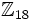# Cyclic group:Z18

View a complete list of particular groups (this is a very huge list!)[SHOW MORE]

## Definition

This group, denoted$C_{18}$,$\Z_{18}$,$\Z/18\Z$, is defined in the following equivalent ways:

1. It is the direct product of cyclic group of order nine and cyclic group of order two.
2. It is the cyclic group of order$18$.

## Arithmetic functions

Function Value Explanation
order 18
exponent 18
Frattini length 2

## GAP implementation

### Group ID

This finite group has order 18 and has ID 2 among the groups of order 18 in GAP's SmallGroup library. For context, there are groups of order 18. It can thus be defined using GAP's SmallGroup function as:

SmallGroup(18,2)

For instance, we can use the following assignment in GAP to create the group and name it$G$:

gap> G := SmallGroup(18,2);

Conversely, to check whether a given group$G$ is in fact the group we want, we can use GAP's IdGroup function:

IdGroup(G) = [18,2]

or just do:

IdGroup(G)

to have GAP output the group ID, that we can then compare to what we want.

### Other descriptions

The group can be defined using GAP's CyclicGroup function:

CyclicGroup(18)